Published © CC BY-NC-ND

PID Light Meter Controller

PID control system of a light meter that measures and regulates the lighting of a lamp.

AdvancedFull instructions providedOver 2 days1,246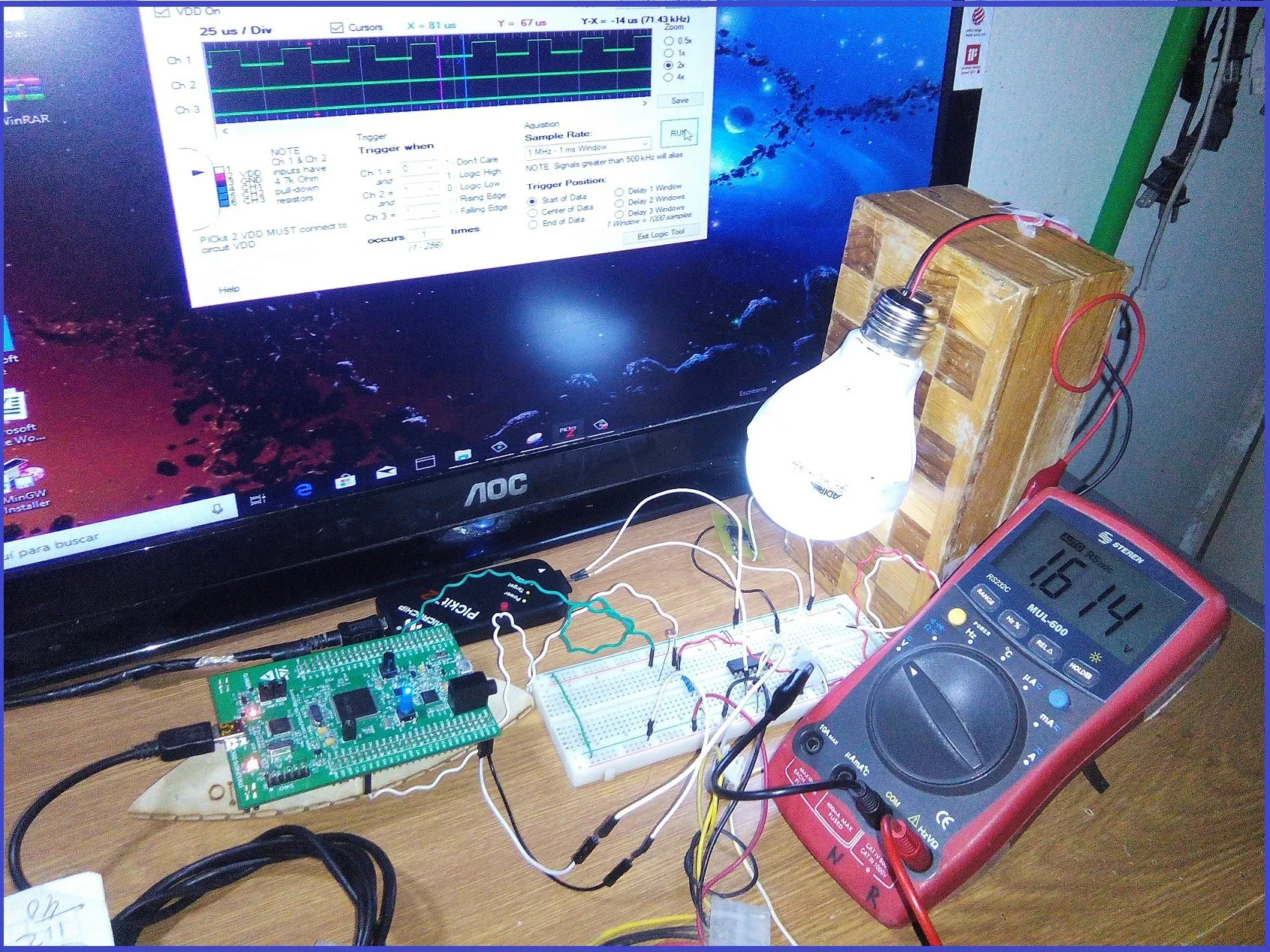Things used in this project

Hardware components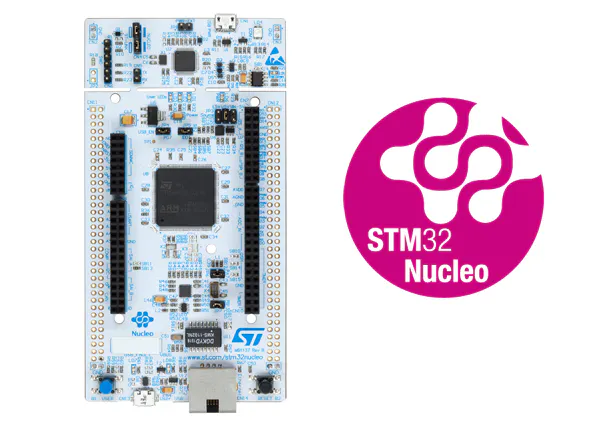ST STM32L4, STM32F7 Nucleo 144 STM32F7
×1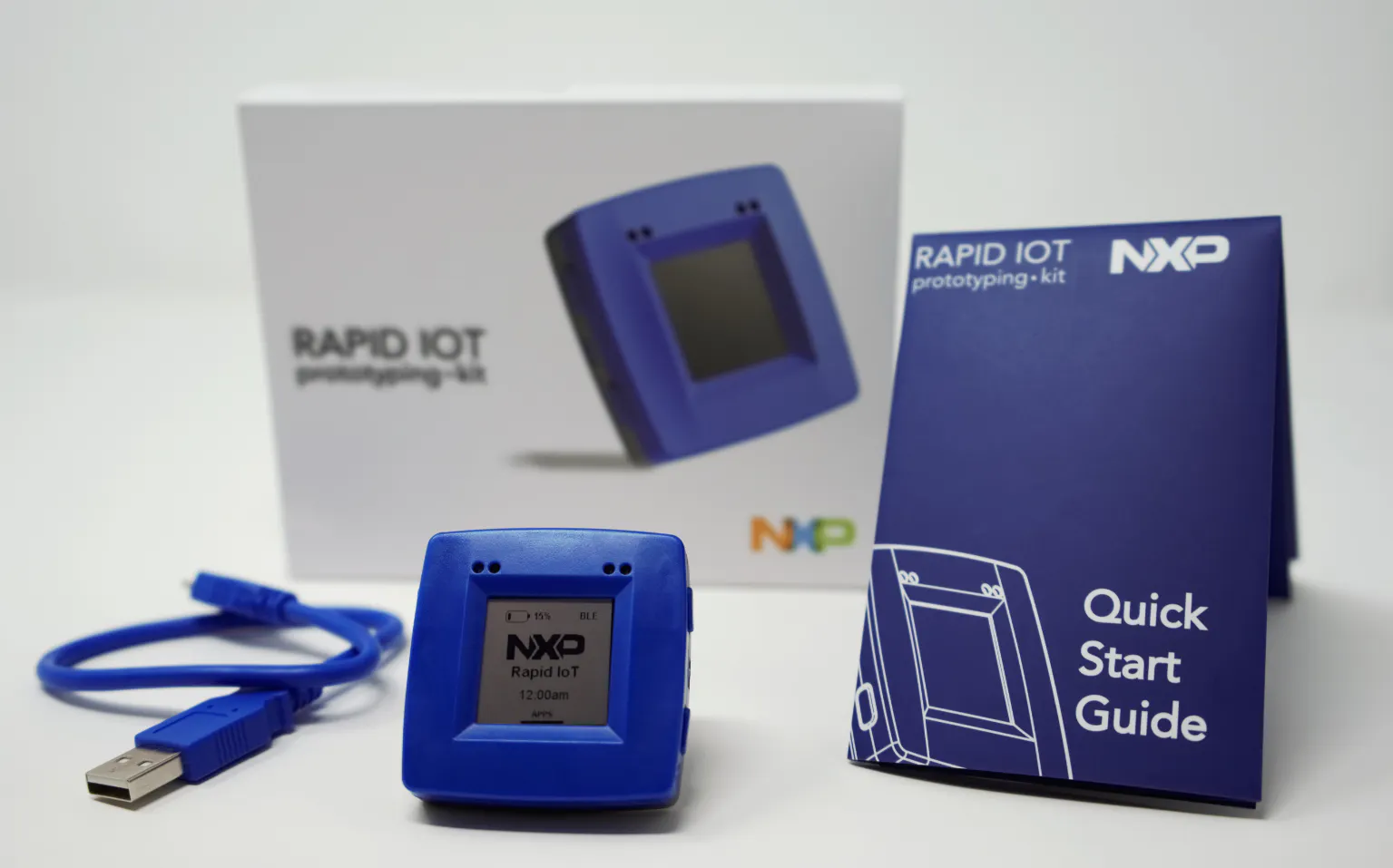NXP Rapid IoT Prototyping Kit
×1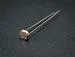Photo resistor
×1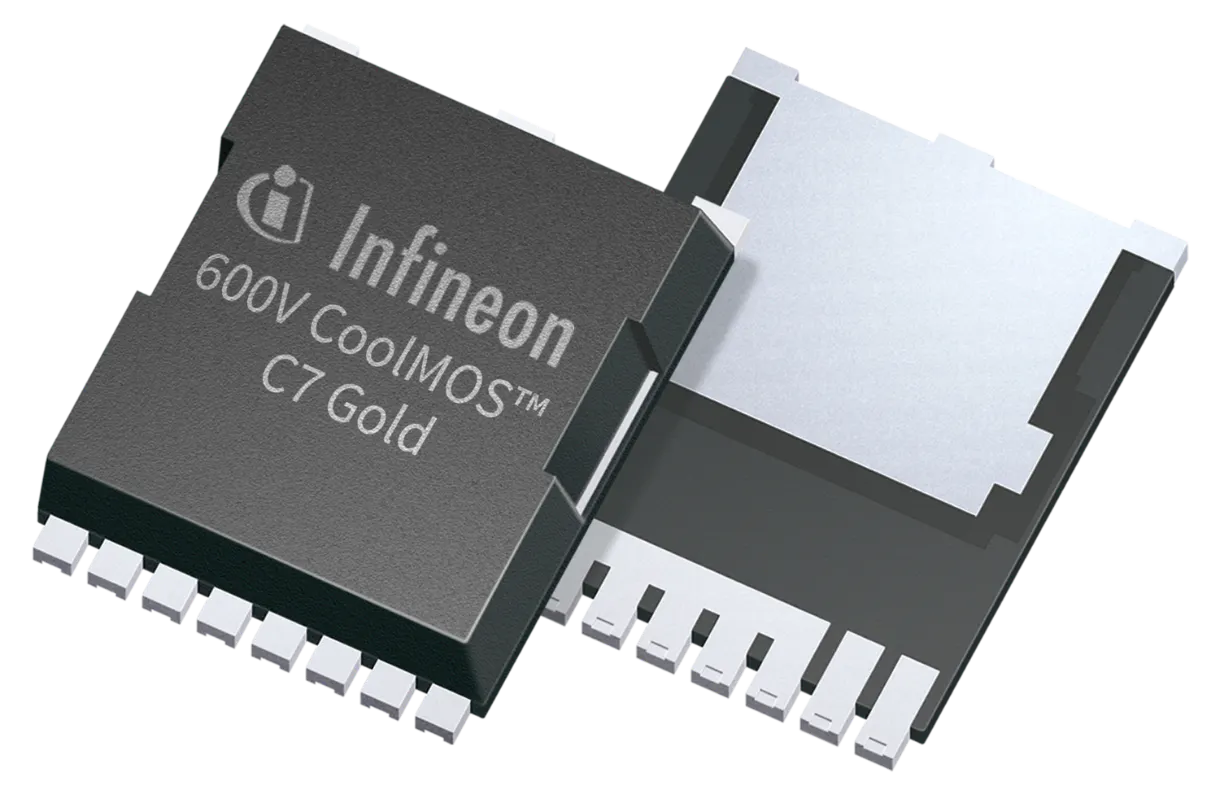Infineon CoolMOS C7 Gold SJ MOSFET
×1
 L293B Driver
×1Resistor 10k ohm
×2Resistor 1k ohm
×1
 Resistor 30 ohm
×10LED (generic)
×10

Software apps and online services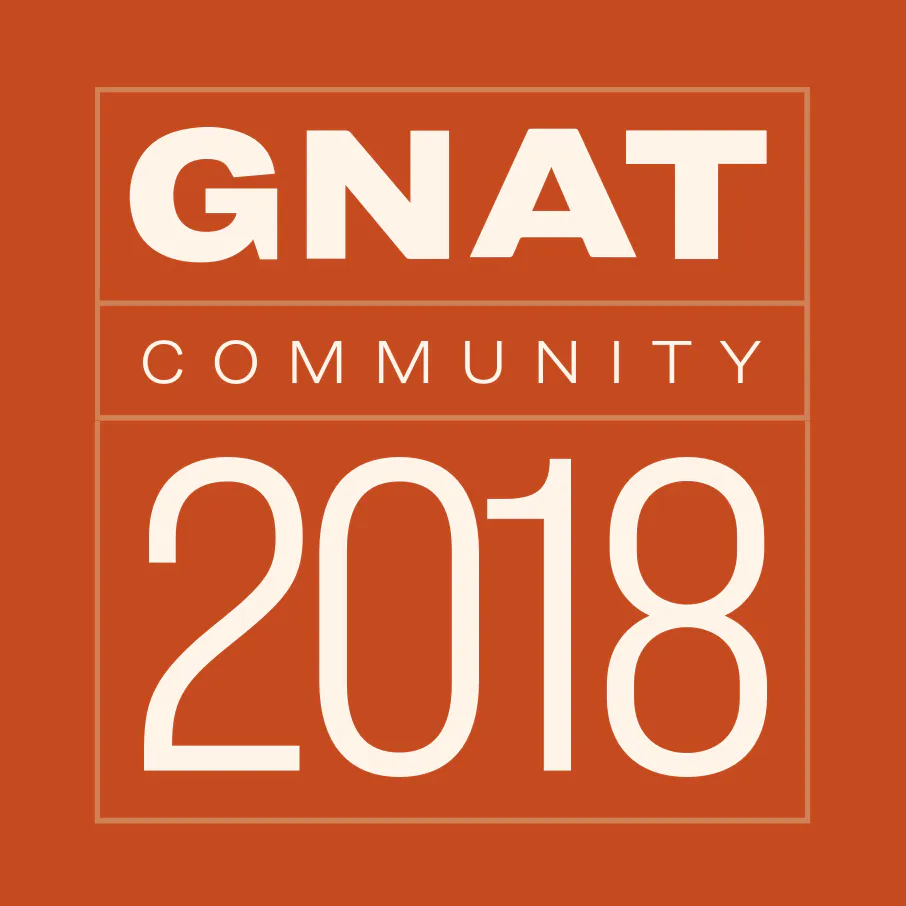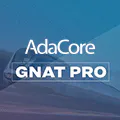NXP Rapid IoT Studio
 PID example By Lowell Cady

Hand tools and fabrication machines

 PICkit 2Digilent Mastech MS8217 Autorange Digital Multimeter

Schematics

Schematic Diagram

Schematic diagram of the project: "PID Light Meter Controller"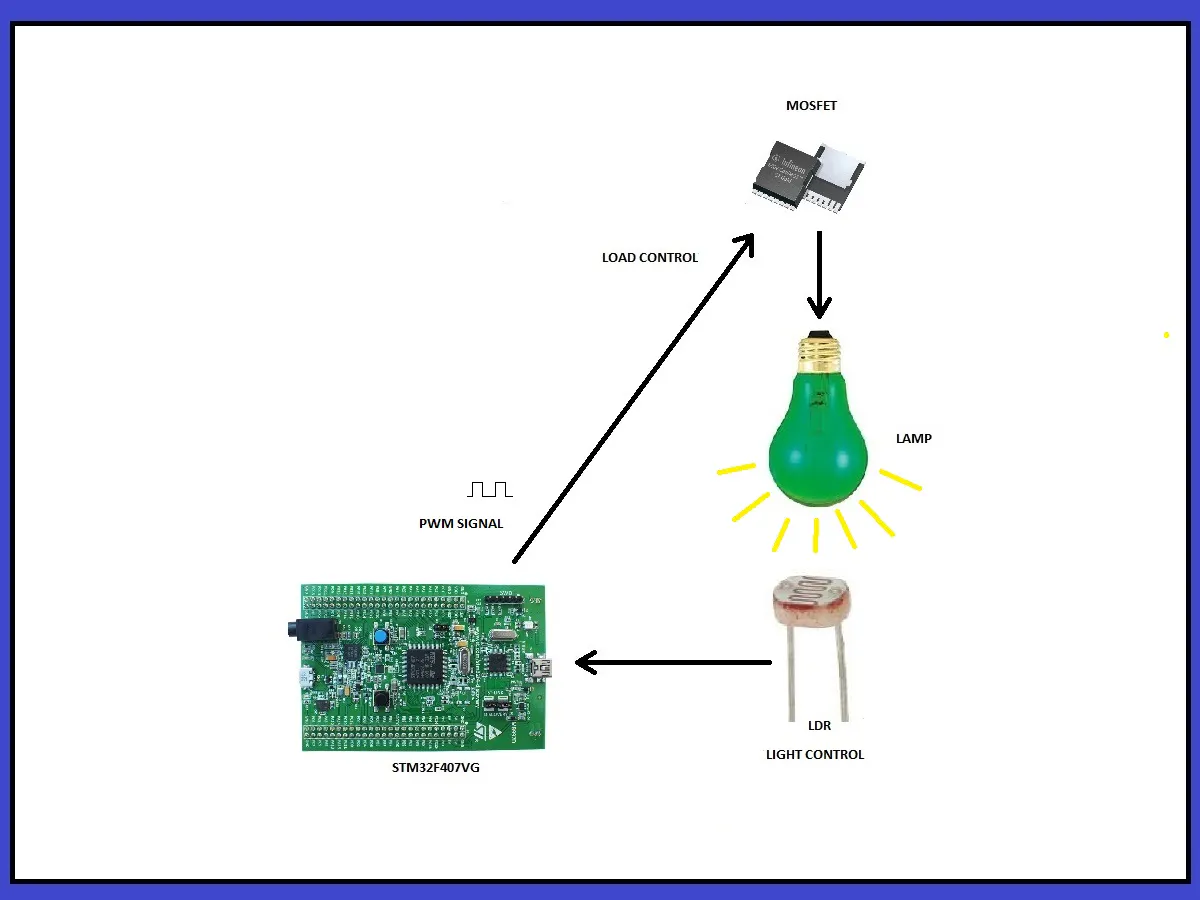Circuit Diagram

Electric diagram of the project: "PID Light Meter Controller"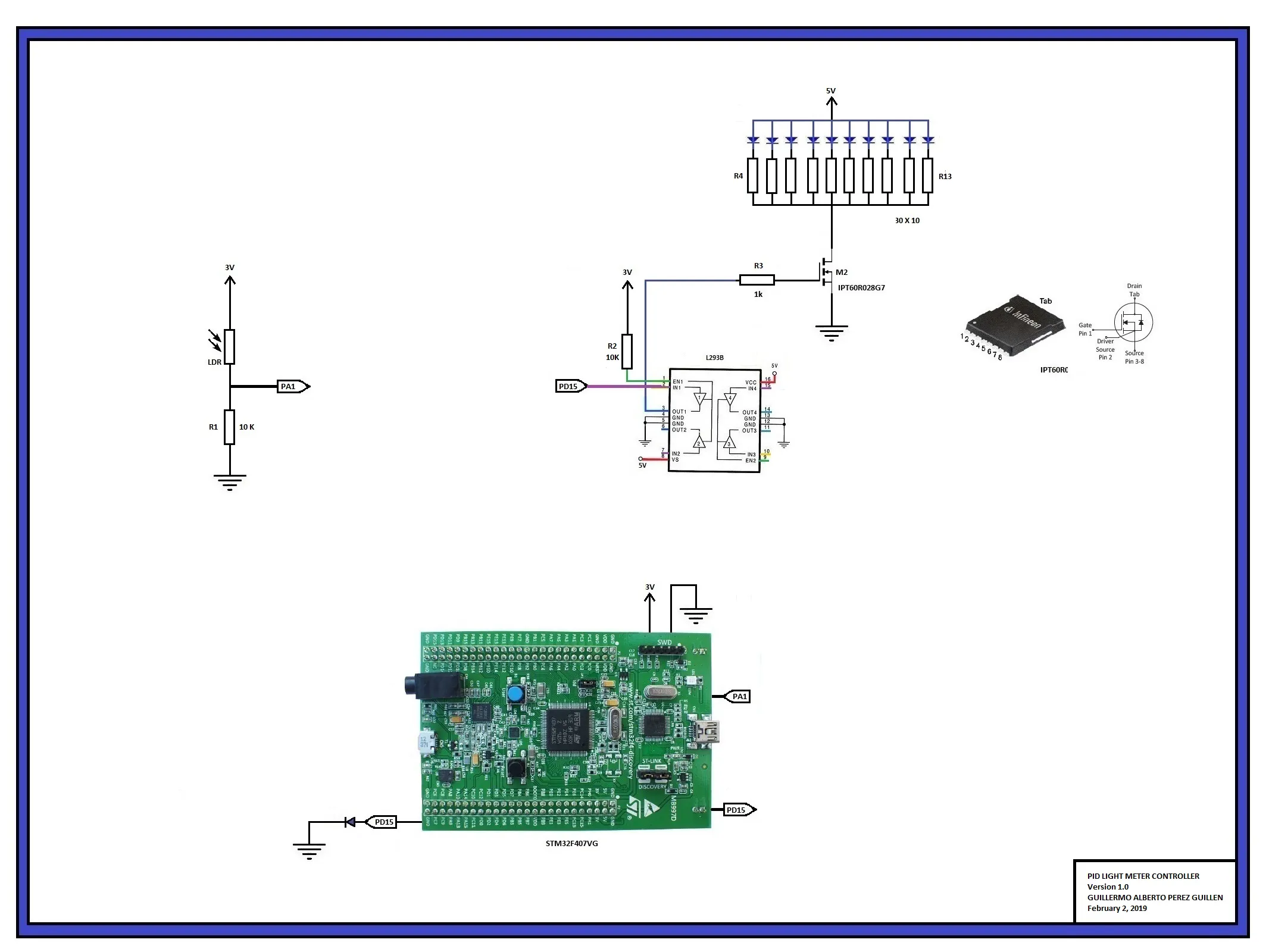Code

--  PID LIGHT METER CONTROLLER
--  Author GUILLERMO ALBERTO PEREZ GUILLEN
--  February 2, 2019
--  This demonstration illustrates the use of:
--  1) PWM PID signal to control a LIGHT on PD15,

with Last_Chance_Handler;  pragma Unreferenced (Last_Chance_Handler);

with STM32.Board;  use STM32.Board;
with STM32.Device; use STM32.Device;
with STM32.PWM;    use STM32.PWM;
with STM32.Timers; use STM32.Timers;

with HAL;          use HAL; --adc library
with STM32.GPIO;   use STM32.GPIO; --adc library

procedure Pid_Light_Control is

Converter     : Analog_To_Digital_Converter renames ADC_1; --adc instruction
Input_Channel : constant Analog_Input_Channel := 1; --adc instruction, channel 1
Input1         : constant GPIO_Point := PA1; --adc instruction, PA1 port

All_Regular_Conversions : constant Regular_Channel_Conversions :=
(1 => (Channel => Input_Channel, Sample_Time => Sample_144_Cycles)); --adc instruction

Raw : UInt32 := 0; --adc instruction

Successful : Boolean; --adc instruction

Selected_Timer : STM32.Timers.Timer renames Timer_4;
--  NOT arbitrary! We drive the on-board LEDs that are tied to the channels
--  of Timer_4 on some boards. Not all boards have this association. If you
--  use a different board, select a GPIO point connected to your selected
--  timer and drive that instead.

Timer_AF : constant STM32.GPIO_Alternate_Function := GPIO_AF_TIM4_2;
--  Note that this value MUST match the corresponding timer selected!

Output_Channel : constant Timer_Channel := Channel_4; -- Blue LED is selected
--  The LED driven by this example is determined by the channel selected.
--  That is so because each channel of Timer_4 is connected to a specific
--  LED in the alternate function configuration on this board. We will
--  initialize all of the LEDs to be in the AF mode. The
--  particular channel selected is completely arbitrary, as long as the
--  selected GPIO port/pin for the LED matches the selected channel.

LED_For : constant array (Timer_Channel) of User_LED :=
(Channel_1 => Green_LED,
Channel_2 => Orange_LED,
Channel_3 => Red_LED,
Channel_4 => Blue_LED);

Requested_Frequency : constant Hertz := 30_000;  -- PWM frequency

Power_Control : PWM_Modulator;

procedure Configure_Analog_Input is --adc instruction
Enable_Clock (Input1); --adc instruction
Configure_IO (Input1, (Mode => Mode_Analog, Resistors => Floating)); -- adc instruction
end Configure_Analog_Input; --adc instruction

begin

Enable_Clock (Converter); --adc instruction

(Mode           => Independent, --adc instruction
Prescalar      => PCLK2_Div_2, --adc instruction
DMA_Mode       => Disabled, --adc instruction
Sampling_Delay => Sampling_Delay_5_Cycles); --adc instruction

Alignment  => Right_Aligned); --adc instruction

Continuous  => False, --adc instruction
Trigger     => Software_Triggered, --adc instruction
Enable_EOC  => True, --adc instruction
Conversions => All_Regular_Conversions); --adc instruction

Enable (Converter); --adc instruction

Configure_PWM_Timer (Selected_Timer'Access, Requested_Frequency);

Power_Control.Attach_PWM_Channel
(Selected_Timer'Access,
Output_Channel,
LED_For (Output_Channel),
Timer_AF);

Power_Control.Enable_Output;

declare
Value     : Percentage;
Raw1      : Long_Float;
--      setpoint  : constant := 1480.0; -- 1.084 volts approx - 43 lx
setpoint  : constant := 2067.0; -- 1.514 volts approx - 86 lx
error     : Long_Float := 0.0;
output    : Long_Float;
integral  : Long_Float := 0.0;
dt        : constant := 0.0005;
Kp        : constant := 0.025;
Ki        : constant := 0.025;

begin
loop
Start_Conversion (Converter); --adc instruction
Poll_For_Status (Converter, Regular_Channel_Conversion_Complete, Successful); --adc instruction
Raw := UInt32 (Conversion_Value (Converter)); -- reading PA1
Raw1 := Long_Float(Raw * 1);
error := (setpoint - Raw1);
integral := (integral + (error*dt));
output := ((Kp*error) + (Ki*integral));
Value := Percentage (output); -- duty cycle value

if Value < 10 then
Power_Control.Set_Duty_Cycle (10);
elsif Value > 90 then
Power_Control.Set_Duty_Cycle (90);

else
Power_Control.Set_Duty_Cycle (Value); -- PWM signal
end if;

delay until Clock + Milliseconds (10); -- slow it down to ease reading
end loop;
end;

end Pid_Light_Control;

pid_light_control,gpr

GNAT project file
with "../../../../../boards/stm32f407_discovery/stm32f407_discovery_full.gpr";

project Pid_Light_Control extends "../../../../../examples/shared/common/common.gpr" is

for Languages use ("Ada");
for Main use ("pid_light_control.adb");
for Source_Dirs use ("src");
for Object_Dir use "obj/" & STM32F407_Discovery_Full.Build;
for Create_Missing_Dirs use "true";

package Builder is
for Global_Configuration_Pragmas use "gnat.adc";
end Builder;

package Compiler renames STM32F407_Discovery_Full.Compiler;

end Pid_Light_Control;

Project repository: "PID Light Meter Controller”

This project contains the project codes: "PID Light Meter Controller" Programming Language: AdaCore

Credits

Guillermo Guillen

29 projects • 30 followers
Communications and Electronics Engineering, and Writer... "Don´t Expect Different Results If Your Habits Are The Same" A Einstein